Next: Cube-Map Up: Parameter Interpolation and Normalization Previous: Parameter Interpolation and Normalization

### Spherical Interpolation

The dot product between two vectors is only equivalent to the cosine of the angle if the two vectors are unit vectors. So the two vectors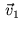and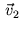should be interpolated in a way that the interpolant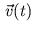is moving uniformly between the two vectors and its length remains one. As  point out this interpolations works on the surface of the unit sphere and therefore it is called spherical interpolation (Formula 3).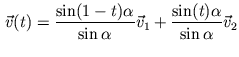(3)

Where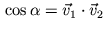. Unfortunately spherical interpolation is not available on current graphics accelerators, only linear interpolation is supported. When using linear interpolation this results in denormalized vectors (Figure 4) and wrong shading intensities.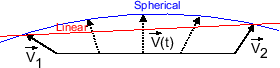Gerald Schröcker 2002-03-21